• Submissions# Innovation in the Engineering Field and Basic Sciences in the Analytical Solution of Nonlinear Partial Differential Equations by New Methods ASM and IAM

Akbari MR1*, Sara Akbari2 and Esmaeil Kalantari3

1Department of Civil Engineering and Chemical Engineering, University of Germany, Germany

1Department of Pharmaceutical Technical Assistant, Dr. Kurt Blindow Vocational School, Germany

1Department of Chemical Engineering, Islamic Azad University, Iran

*Corresponding author:Akbari MR, Department of Civil Engineering and Chemical Engineering, University of Germany, Germany

Submission: September 21, 2022;Published: October 20, 2022

DOI: 10.31031/RDMS.2022.18.000927ISSN: 2576-8840
Volume 18 Issue 1

#### Abstract

In this article, we want to solve an analytical nonlinear partial differential equation that it is very applicable in all fields of engineering and basic sciences. This kind of nonlinear partial equations is used extensively in most engineering fields including chemical, mechanical, civil, electrical, and other engineering. As all experts know most of engineering actual systems behavior in practical are nonlinear process and analytical scrutiny these nonlinear problems (especially nonlinear analytical solution of partial equations) are very difficult or sometimes impossible. Our purpose is to enhance the ability of solving the mentioned nonlinear partial differential equations at engineering fields with a simple and innovative approach which entitled ‘’Akbari-Sara’s Method’’ or ‘’ASM’’ and ‘’Integral Akbari-Method’’ or ‘’IAM’’.

Keywords: Akbari-Sara’s Method (ASM); Integral Akbari Method (IAM); Nonlinear partial differential equation, Analytical solution, Eigenvalues

#### Introduction

Partial nonlinear differential equations which arise in real-world physical problems are often too complicated to be solved exactly [1,2]. And even if an exact solution is obtainable, the required calculations may be too complicated to be practical, or it might be difficult to interpret the outcome. In fact, solving nonlinear equations can guide researchers to comprehend the physical phenomena deeply and sometimes leads them to investigate some facts which are not easily understood through common observations [3-5]. On the basis of the above explanations, here we aim to discuss nonlinear partial differential and the behavior of the physical its. In this literature, we have a governing equation for studying nonlinear behavior in engineering processes and solving it analytically with a new approaches like AGM, Akbari Ganji Method [6-13]. This method is called the abbreviation ASM (Akbari Sara’s Method), which can easily solve all nonlinear problems in case analytical and is very simple and flexible. Other methods compared to ASM do not have this ability to gain the solution of the presented problem in high precision and accuracy so nonlinear differential equations such as the presented problem in this case study should be solved by utilizing new approaches. In recent years, analytical methods in solving nonlinear differential equations have been presented and created by Mohammadreza Akbari, these methods are called AKLM  (Akbari Kalantri Leila Method in August 2020), ASM [15,16] (Akbari Sara’s Method in August 2019) and AYM  (Akbari Yasna’s Method in April 2020), MR.AM (Mohammad Reza Akbari Method in November 2020) and IAM (Integral Akbari Method in November February 2021).

Application

We consider an unsteady state of nonlinear partial differential equation with constant physical coefficients that depends on the engineering process. The constant values α ,β ,η,ε ,ρ , ko, p,u1,u2,uo depend on what field of engineering we want to solve this equation. For example, these constant values in mass transfer, heat and fluid transfer, electrical circuits, as well as in beam-column equations are defined respectively in chemical engineering, mechanical engineering, civil engineering and electrical engineering, economical and all engineering fields and basics sciences.

The governing nonlinear partial differential equation of engineering fields is expressed as follows:Boundary and initial condition:i. Solving partial nonlinear differential equation by ASM (Akbari-Sara’s Method)

We separate equation Eq. (1) from steady and unsteady state as follows: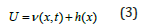After separation, the result is given by the following two differential equations for p = 2 :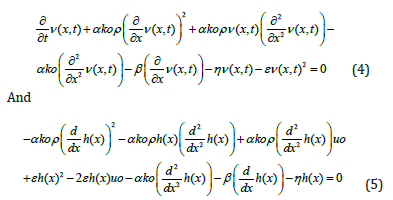Using the separation equation ν (x,t) =φ (x) ⋅ z(t) in Eq. (4) as follows: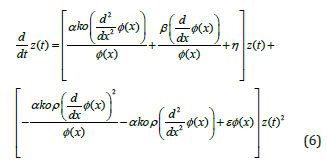The coefficient z(t) in the Eq. (6) can derive the following differential equation for calculating eigenvalues λ and its functionSolve Eq. (7) as follows:And boundary conditions:The constant coefficients _C1− _C2 are obtained from Eq. (8) and given the homogeneous boundary condition with forming the coefficients matrix for the homogeneous equation as follow:Analytical Solution of Nonlinear Differential Equation in Steady State Eq. (5) by the boundary conditions h(0) = u1;h(L) = u2 is obtained by ASM method as follows: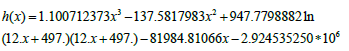Using the orthogonality of the modes of the function φ(x) in Eq. (6), we can obtain the followingAfter substituted the physical values Eqs. (12) and the eigenvalue function Eqs. (10,11), and solving it by ASM method Eq.(13), the following is obtained:ii. Comparing the achieved solutions by numerical method and ASM (Akbari Sara’s Method)

(Figure 1) And finally the solution of the nonlinear partial differential Eq. (1) is solved analytically by ASM method as follow:The diagram of the place-time is as follows (Figure 2).

Figure 1:A comparison between ASM and Numerical solution.Figure 2:Figures of ASM solution.iii. Solving partial nonlinear differential equation by IAM (Integral Akbari Method)

The governing nonlinear partial differential equation of engineering fields is expressed as follows:Boundary and initial condition:IAM solution process (Integral Akbari Method) The following values have been used for the physical parameters of Eqs. (12), the output answer the Eq. (16) according to boundary conditions Eqs. (17) by IAM method is obtained as follows: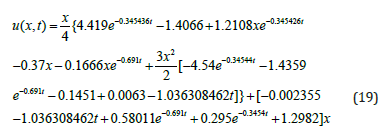We compare solution of IAM method of Eq. (19) with the numerical method (Runge-Kutte 4th), for the different times as shown below (Figure 3).

Figure 3:A comparison between IAM and Numerical solution and contour graphs.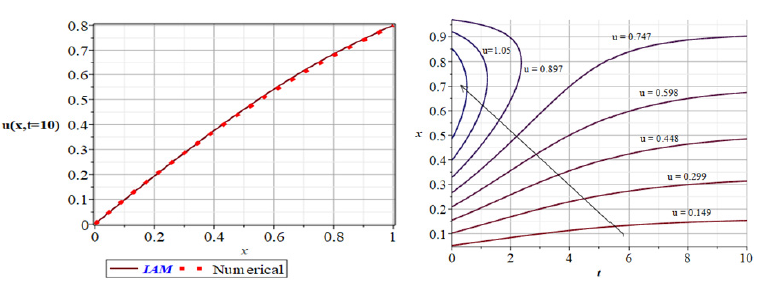#### Conclusion

In this paper, one complicated nonlinear partial differential equations have been introduced and analyzed completely by Akbari-Sara’s Method (ASM), Integral Akbari-Method (IAM) and the obtained results have been compared with Numerical Method (Runge-Kutte 4th). A modern methods (ASM, IAM) for solving all kinds of complicate nonlinear partial differential equations in engineer field and basic science which can be PDEs or ODEs has been presented.

1. He JH (1998) Approximate solution for nonlinear differential equations with convolution product nonlinearities. Comput Math Appl Mech Eng 167: 69-73.
2. He JH (1999) Variational iteration method: a kind of nonlinear analytical technique: some examples. Int J Non-Linear Mech 344: 699.
3. Wu X, Lu X (2003) Tendon model for nonlinear analysis of externally prestressed concrete structures. J Struct Eng 129(1): 96-104.
4. Ji-Huan He, Xu-Hong Wu (2006) Exp-function method for nonlinear wave equations. Chaos, Solitos & Fractals 30(3): 700-708.
5. Xu-Hong WU, Ji-Huan He (2007) Solitary solutions, periodic solutions and compacton-like solutions using the Exp-function method. Computers & Mathematics with Applicatios 54(7-8): 966-986.
6. Akbari MR, Sara Akbari, Esmaeil Kalantari, Ganji DD (2020) Akbari-Ganji’s method “AGM” to chemical reactor design for non-isothermal and non-adiabatic of mixed flow reactors. Journal of Chemical Engineering and Materials Science 11(1): 1-9.
7. Akbari MR, Ganji DD, Nimafar M (2014) Significant progress in solution of nonlinear equations at displacement of structure and heat transfer extended surface by new AGM approach. Frontiers of Mechanical Engineering Journal 9: 390-401.
8. Rostami AK, Akbari MR, Ganji DD, Heydari S (2014) Investigating jeffery-hamel flow with high magnetic field and nanoparticle by HPM and AGM. Cent Eur J Eng 4(4): 357-370.
9. Akbari MR, Ganji DD, Majidian A, Ahmadi AR (2014) Solving nonlinear differential equations of Vanderpol, Rayleigh and Duffing by AGM. Frontiers of Mechanical Engineering 9: 177-190.
10. Ganji DD, Akbari MR, Goltabar AR (2014) Dynamic vibration analysis for non-linear partial differential equation of the beam - columns with shear deformation and rotary inertia by AGM. Development and Applications of Oceanic Engineering (DAOE).
11. Akbari MR, Ganji DD, Ahmadi AR, Sayyid H, Hashemi K (2014) Analyzing the Nonlinear vibrational wave differential equation for the simplified model of Tower Cranes by (AGM). Frontiers of Mechanical Engineering 9(1): 58-70.
12. Akbari MR, Nimafar M, Ganji DD, Akbarzade MM (2014) Scrutiny of non-linear differential equations Euler Bernoulli beam with large rotational deviation by AGM. Frontiers of Mechanical Engineering 9: 402-408.
13. Akbari MR, Ahmadi AR. Nonlinear dynamic in engineering by akbari-Ganji's Method.
14. Akbari MR, Sara Akbari, Esmaeil Kalantari (2020) Chemical reactors catalyst bed and analytical solution of complicated partial nonlinear differential equations by new approach AKLM.
15. Akbari MR, Sara Akbari, Esmaeil Kalantari (2020) A study about exothermic chemical reactor by ASM approach strategy. Determinations Named Nontechnical 2(1): 1-3.
16. Akbari MR, Sara Akbari, Esmaeil Kalantari (2021) Analyzing the nonlinear mass and heat balance equations on the reactor catalyst bed by ASM method. IJO -International Journal of Mechanical and Civil Engineering 4(1).
17. Akbari MR, Sara Akbari, Esmaeil Kalantari (2021) Analytical solution of schrödinger nonlinear partial differential equation in the quantum physic by new approach AYM. IJRDO - Journal of Mechanical and Civil Engineering 7(1).

We at Crimson Publishing are a group of people with a combined passion for science and research, who wants to bring to the world a unified platform where all scientific know-how is available read more...